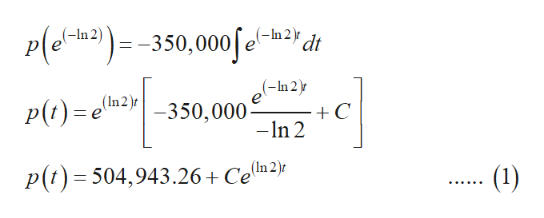# The population of mosquitoes in a certain area increases at a rate proportional to the current population, and in the absence of other factors, the population doubles each week. There are  400,000 mosquitoes in the area initially, and predators (birds, bats, and so forth) eat  50,000 mosquitoes/day. Determine the population of mosquitoes in the area at any time. (Note that the variable t represents days.)

Question
131 views

The population of mosquitoes in a certain area increases at a rate proportional to the current population, and in the absence of other factors, the population doubles each week. There are  400,000 mosquitoes in the area initially, and predators (birds, bats, and so forth) eat  50,000 mosquitoes/day. Determine the population of mosquitoes in the area at any time. (Note that the variable t represents days.)

check_circle

Step 1

Calculation:

Since the population of mosquitoes in a certain area increases at a rate proportional to the current population, assume a differential equation in the form (dp/dt) =kp, where k is the constant. And the solution of the above differential equation will be  p(t) = Cekt.

Since it is given that the population of mosquitoes doubles in each week, p(1) = 2p(0).

That is, Cek = 2 Ce0

On simplification, k = ln(2).

It is also given that 50,000 mosquitoes per day are eaten by predators. Then for a week, 350,000 mosquitoes are eaten by them.

Then the population of mosquitoes including the predator interaction can be modelled as follows.

Step 2

Solve the above equation as follows.help_outlineImage TranscriptioncloseP(a:) -350,000feyd (-In (-In2)r 'dt (-In2) (In2)r -350,000 +C -In 2 (In (1) p(t) 504,943.26+ Cem2} fullscreen
Step 3

Since the initial population is given as 400000, the value of p(0) ...

### Want to see the full answer?

See Solution

#### Want to see this answer and more?

Solutions are written by subject experts who are available 24/7. Questions are typically answered within 1 hour.*

See Solution
*Response times may vary by subject and question.
Tagged in

### Math